# Review 8 5 Exponential Functions Quadratic Functions Linear

• Slides: 33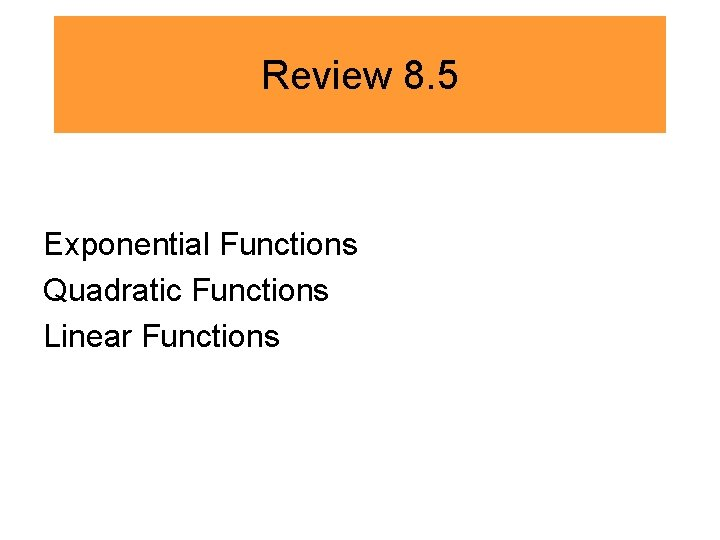Review 8. 5 Exponential Functions Quadratic Functions Linear FunctionsIdentifying from an equation: Linear Quadratic Exponential Has an x with no exponent. Has an x 2 in the equation. Has an x as the exponent. y = 5 x + 1 y = ½x 2 x + 3 y = 6 y = 2 x 2 + 3 x – 5 y = x 2 + 9 x 2 + 4 y = 7 y = 3 x + 1 y = 52 x 4 x + y = 13Examples: • LINEAR, QUADRATIC or EXPONENTIAL? a)y = 6 x + 3 b)y = 7 x 2 +5 x – 2 c)9 x + 3 = y d)42 x = 8Examples: • LINEAR, QUADRATIC or EXPONENTIAL? a)y = 6 x + 3 EXPONENTIAL b)y = 7 x 2 +5 x – 2 QUADRATIC c)9 x + 3 = y LINEAR d)42 x = 8 EXPONENTIAL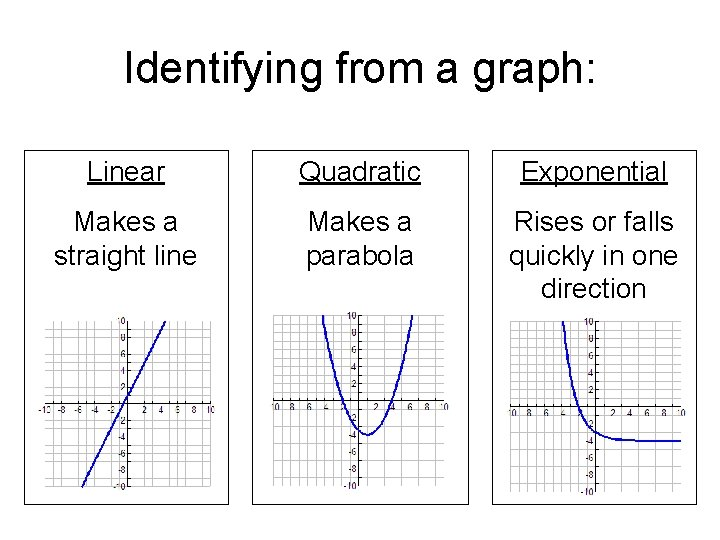Identifying from a graph: Linear Quadratic Exponential Makes a straight line Makes a parabola Rises or falls quickly in one directionLINEAR, QUADRATIC or EXPONENTIAL? a) b) c) d)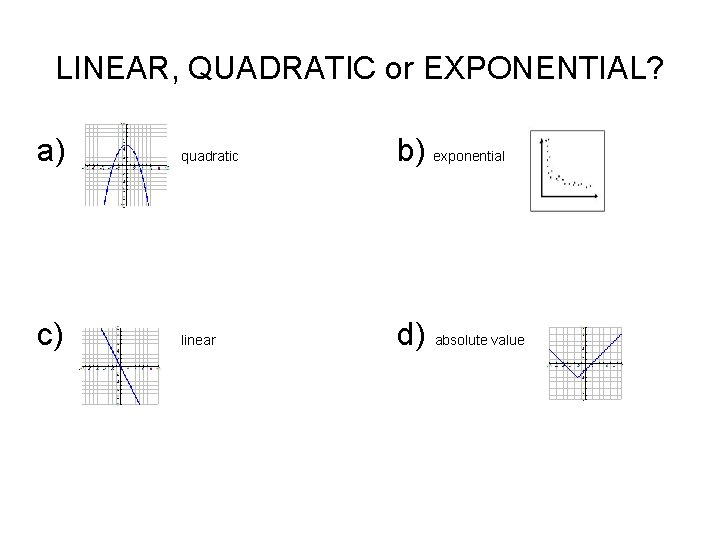LINEAR, QUADRATIC or EXPONENTIAL? a) quadratic b) exponential c) linear d) absolute value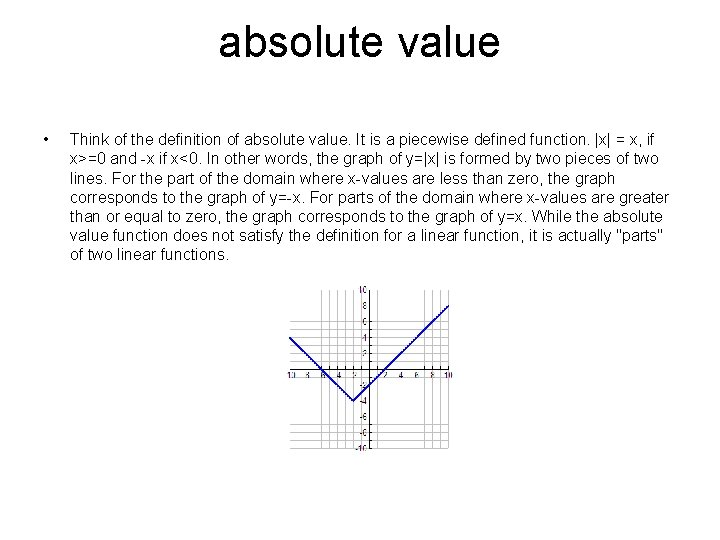absolute value • Think of the definition of absolute value. It is a piecewise defined function. |x| = x, if x>=0 and -x if x<0. In other words, the graph of y=|x| is formed by two pieces of two lines. For the part of the domain where x-values are less than zero, the graph corresponds to the graph of y=-x. For parts of the domain where x-values are greater than or equal to zero, the graph corresponds to the graph of y=x. While the absolute value function does not satisfy the definition for a linear function, it is actually "parts" of two linear functions.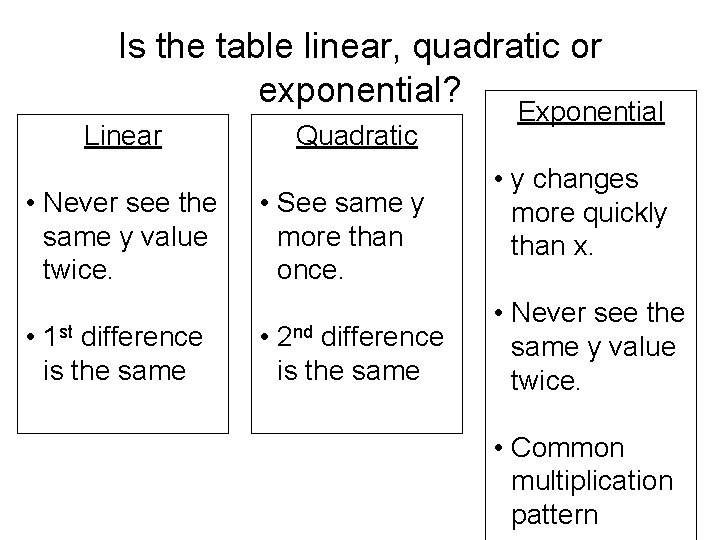Is the table linear, quadratic or exponential? Linear • Never see the same y value twice. • 1 st difference is the same Quadratic • See same y more than once. • 2 nd difference is the same Exponential • y changes more quickly than x. • Never see the same y value twice. • Common multiplication patternConcept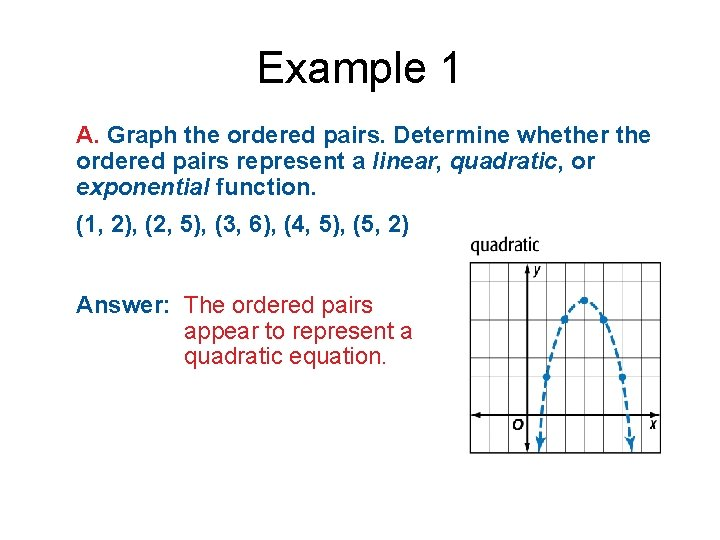Example 1 A. Graph the ordered pairs. Determine whether the ordered pairs represent a linear, quadratic, or exponential function. (1, 2), (2, 5), (3, 6), (4, 5), (5, 2) Answer: The ordered pairs appear to represent a quadratic equation.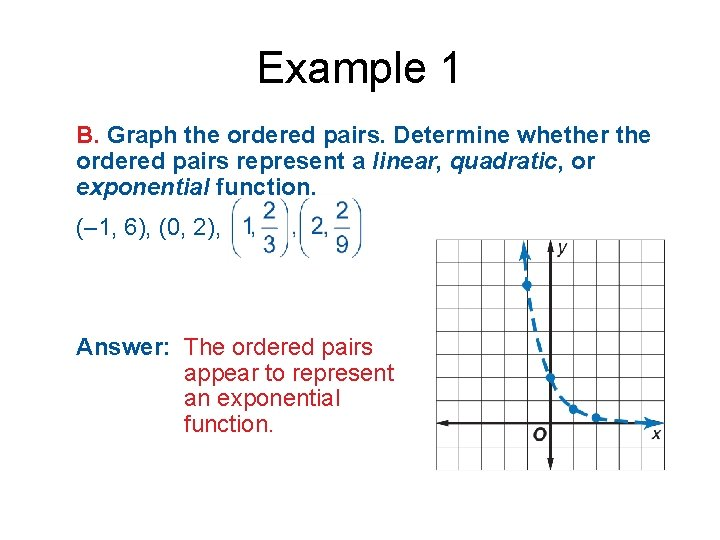Example 1 B. Graph the ordered pairs. Determine whether the ordered pairs represent a linear, quadratic, or exponential function. (– 1, 6), (0, 2), Answer: The ordered pairs appear to represent an exponential function.Example 1 A. Graph the set of ordered pairs. Determine whether the ordered pairs represent a linear, quadratic, or exponential function. (– 2, – 6), (0, – 3), (2, 0), (4, 3) A. linear B. quadratic C. exponentialExample 1 B. Graph the set of ordered pairs. Determine whether the ordered pairs represent a linear, quadratic, or exponential function. (– 2, 0), (– 1, – 3), (0, – 4), (1, – 3), (2, 0) A. linear B. quadratic C. exponential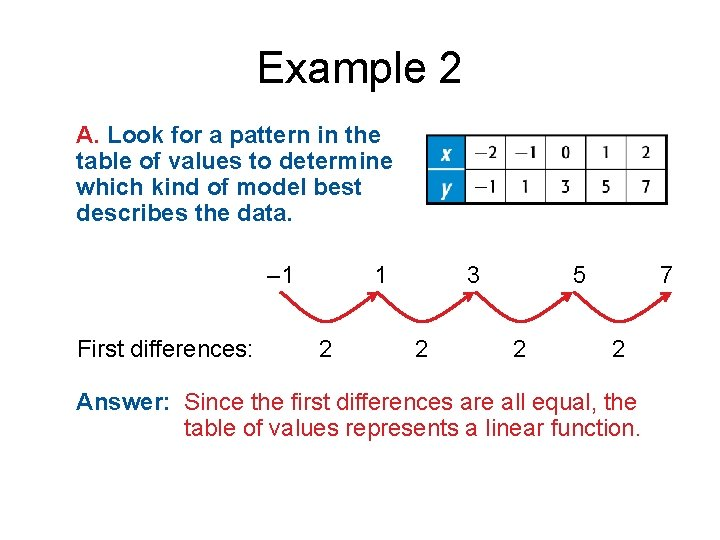Example 2 A. Look for a pattern in the table of values to determine which kind of model best describes the data. – 1 First differences: 1 2 3 2 5 2 7 2 Answer: Since the first differences are all equal, the table of values represents a linear function.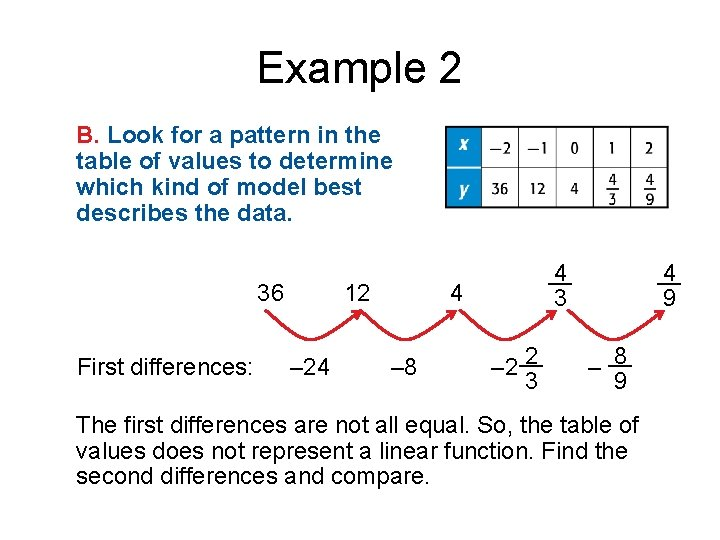Example 2 B. Look for a pattern in the table of values to determine which kind of model best describes the data. 36 First differences: 12 – 24 __ 4 4 – 8 __ 4 3 2 – 2 __ 3 9 8 – __ 9 The first differences are not all equal. So, the table of values does not represent a linear function. Find the second differences and compare.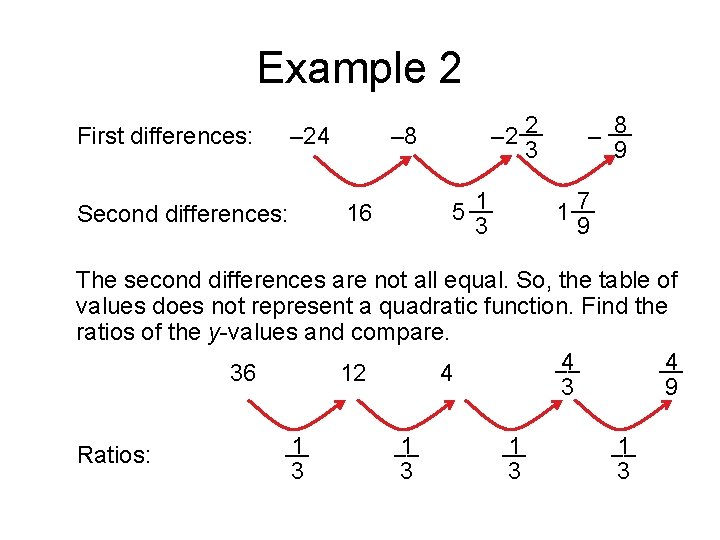Example 2 – 24 First differences: – 8 1 5 __ 3 16 Second differences: 2 – 2 __ 3 8 – __ 9 7 1 __ 9 The second differences are not all equal. So, the table of values does not represent a quadratic function. Find the ratios of the y-values and compare. __ __ 4 4 36 12 4 3 9 Ratios: __ 1 3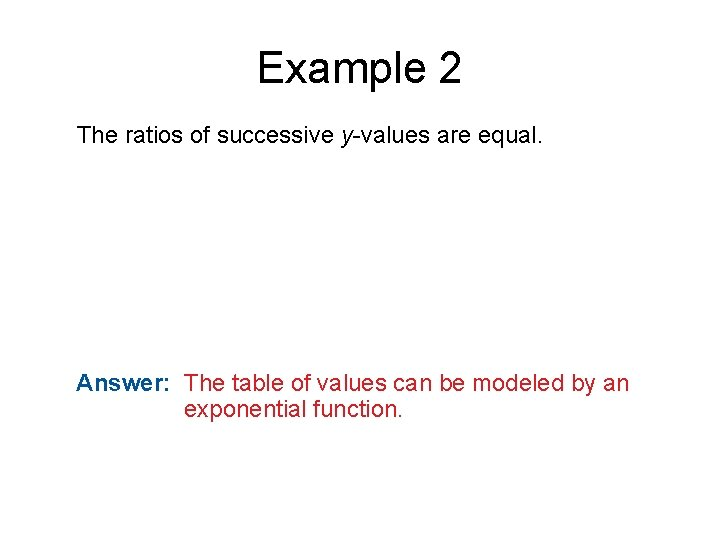Example 2 The ratios of successive y-values are equal. Answer: The table of values can be modeled by an exponential function.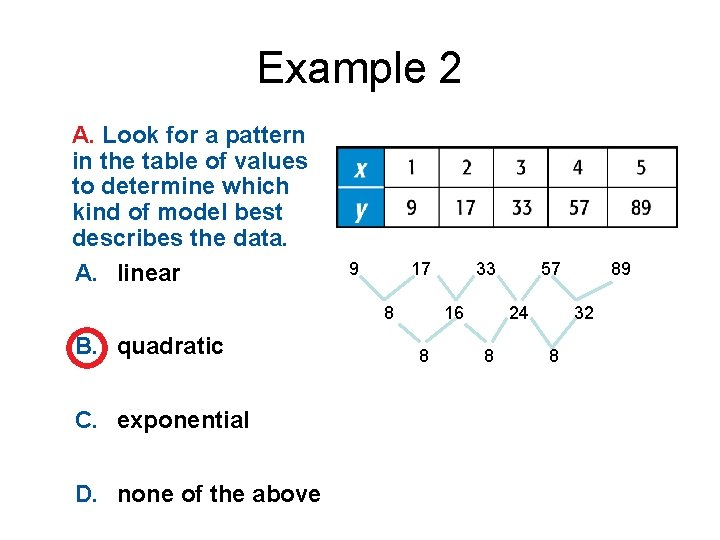Example 2 A. Look for a pattern in the table of values to determine which kind of model best describes the data. A. linear 9 17 8 B. quadratic C. exponential D. none of the above 33 16 8 57 24 8 89 32 8Example 2 B. Look for a pattern in the table of values to determine which kind of model best describes the data. A. linear B. quadratic C. exponential D. none of the above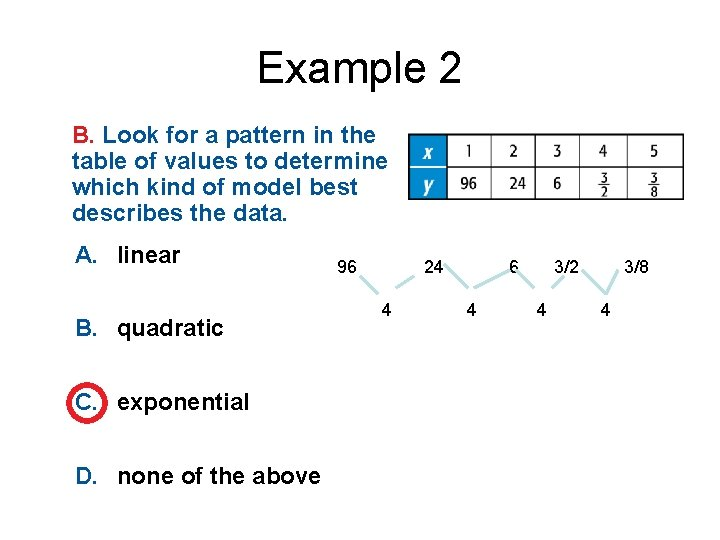Example 2 B. Look for a pattern in the table of values to determine which kind of model best describes the data. A. linear B. quadratic C. exponential D. none of the above 96 24 4 6 4 3/2 4 3/8 4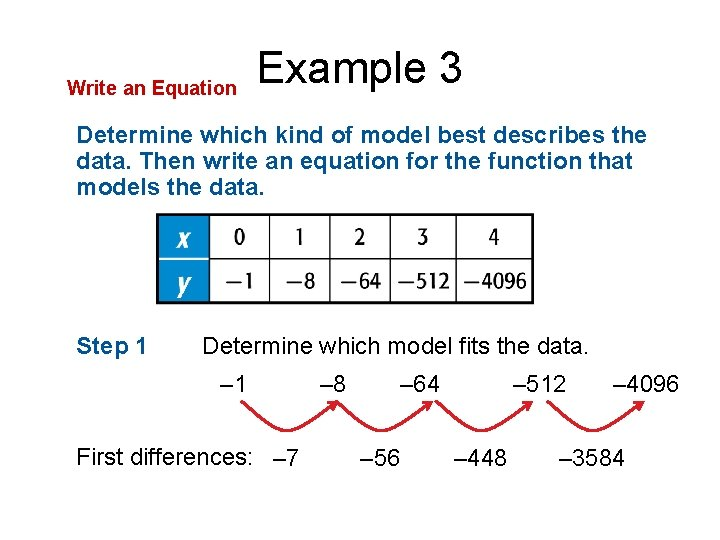Write an Equation Example 3 Determine which kind of model best describes the data. Then write an equation for the function that models the data. Step 1 Determine which model fits the data. – 1 First differences: – 7 – 8 – 64 – 56 – 512 – 448 – 4096 – 3584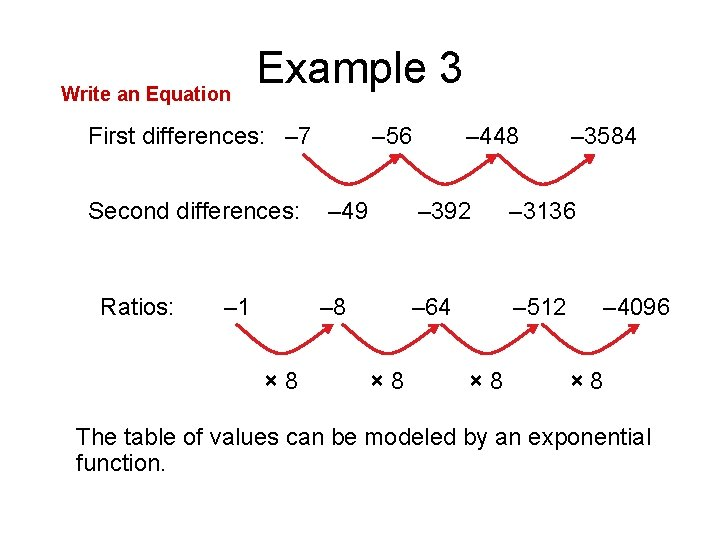Write an Equation Example 3 First differences: – 7 Second differences: Ratios: – 1 – 56 – 49 – 8 × 8 – 448 – 3584 – 392 – 3136 – 64 – 512 × 8 – 4096 × 8 The table of values can be modeled by an exponential function.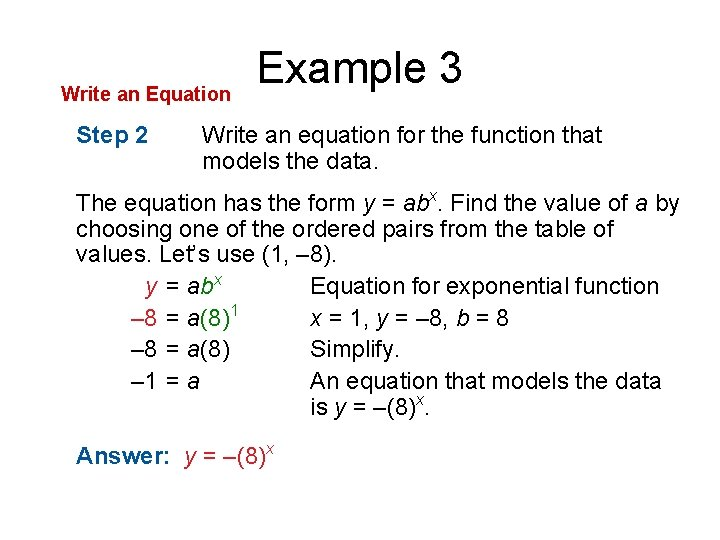Write an Equation Step 2 Example 3 Write an equation for the function that models the data. The equation has the form y = abx. Find the value of a by choosing one of the ordered pairs from the table of values. Let’s use (1, – 8). y = abx Equation for exponential function – 8 = a(8)1 x = 1, y = – 8, b = 8 – 8 = a(8) Simplify. – 1 = a An equation that models the data is y = –(8)x. Answer: y = –(8)x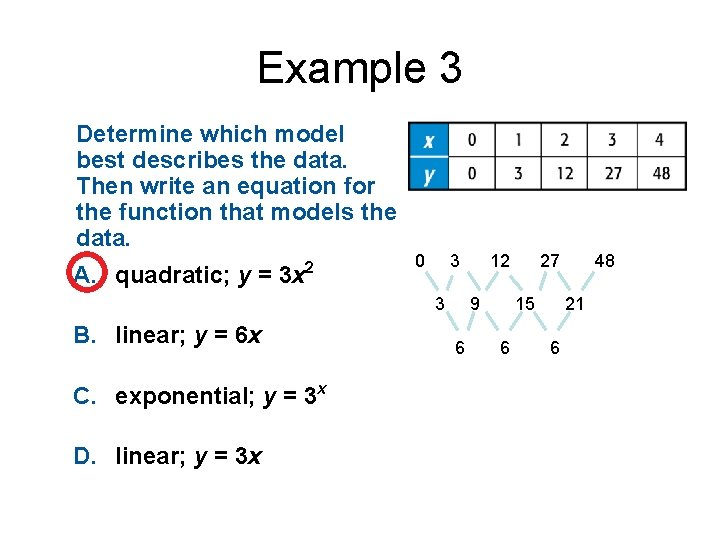Example 3 Determine which model best describes the data. Then write an equation for the function that models the data. A. quadratic; y = 3 x 2 0 3 3 B. linear; y = 6 x C. exponential; y = 3 x D. linear; y = 3 x 12 9 6 27 15 6 48 21 6Write an Equation for a Real-World Situation Example 4 KARATE The table shows the number of children enrolled in a beginner’s karate class for four consecutive years. Determine which model best represents the data. Then write a function that models that data.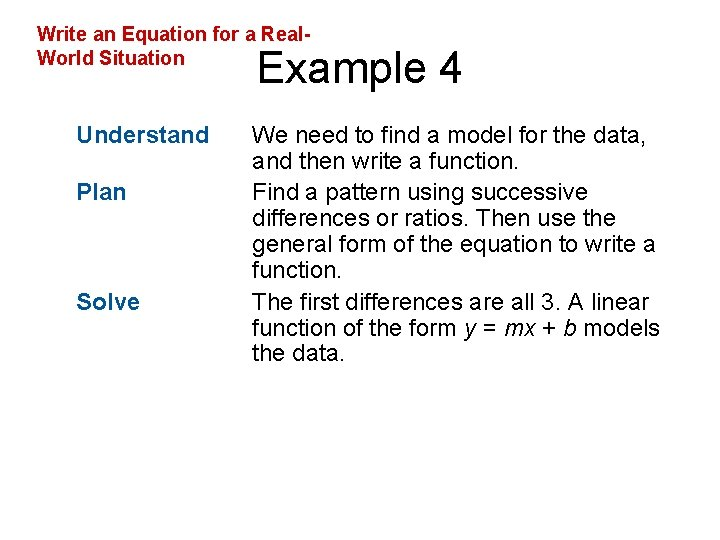Write an Equation for a Real. World Situation Example 4 Understand Plan Solve We need to find a model for the data, and then write a function. Find a pattern using successive differences or ratios. Then use the general form of the equation to write a function. The first differences are all 3. A linear function of the form y = mx + b models the data.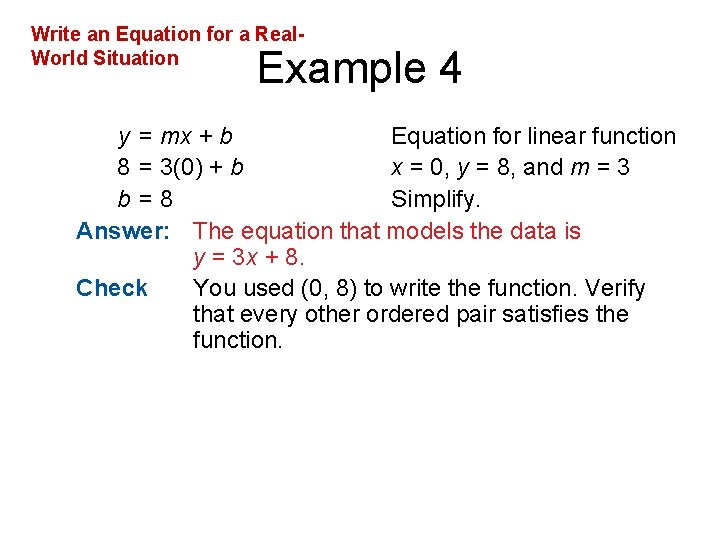Write an Equation for a Real. World Situation Example 4 y = mx + b Equation for linear function 8 = 3(0) + b x = 0, y = 8, and m = 3 b =8 Simplify. Answer: The equation that models the data is y = 3 x + 8. Check You used (0, 8) to write the function. Verify that every other ordered pair satisfies the function.Example 4 WILDLIFE The table shows the growth of prairie dogs in a colony over the years. Determine which model best represents the data. Then write a function that models the data. A. linear; y = 4 x + 4 B. quadratic; y = 8 x 2 C. exponential; y = 2 ● 4 x D. exponential; y = 4 ● 2 x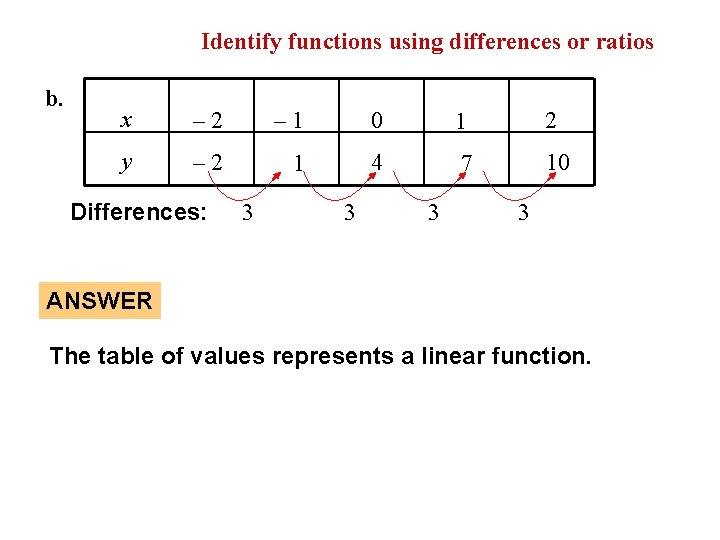EXAMPLE 2 b. Identify functions using differences or ratios x – 2 – 1 0 1 2 y – 2 1 4 7 10 Differences: 3 3 ANSWER The table of values represents a linear function.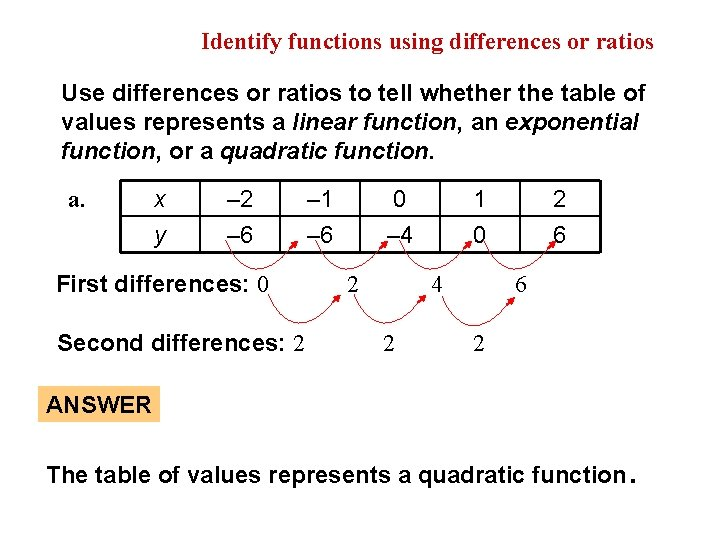EXAMPLE 2 Identify functions using differences or ratios Use differences or ratios to tell whether the table of values represents a linear function, an exponential function, or a quadratic function. a. x y – 2 – 6 First differences: 0 Second differences: 2 – 1 – 6 0 – 4 2 1 0 4 2 2 6 6 2 ANSWER The table of values represents a quadratic function .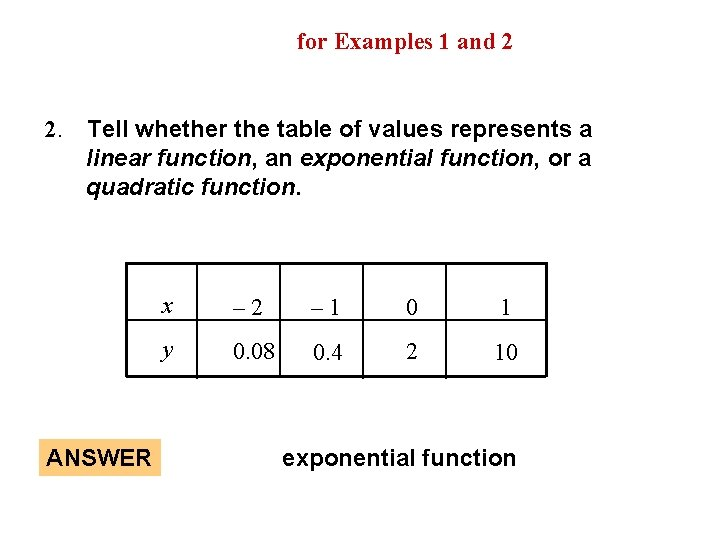GUIDED PRACTICE for Examples 1 and 2 2. Tell whether the table of values represents a linear function, an exponential function, or a quadratic function. ANSWER x – 2 – 1 0 1 y 0. 08 0. 4 2 10 exponential function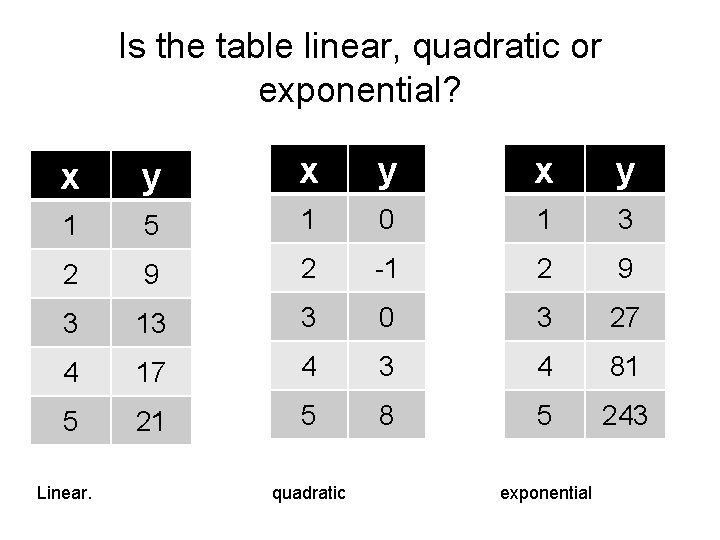Is the table linear, quadratic or exponential? x y x y 1 5 1 0 1 3 2 9 2 -1 2 9 3 13 3 0 3 27 4 17 4 3 4 81 5 21 5 8 5 243 Linear. quadratic exponential